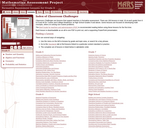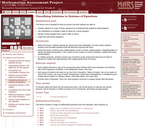Updating search results...

# 2 Results

View
Selected filters:
• Mathematics Assessment Resource Service, University of NottinghamUnrestricted Use
CC BY
Rating
0.0 stars

This is a lesson plan designed to allow students to recall and use the properties of exponents to generate equivalent numeric expressions, identify the appropriate property to use and apply it correctly, and check the numerical value of an expression involving exponents without using a calculator. There is a fun matching activity for students at the end of the lesson to allow students to practice what they have learned and for the teacher to assess their learning by listening to and watching the students work and discuss strategy with each other.

Subject:
Mathematics
Material Type:
Activity/Lab
Diagram/Illustration
Lesson Plan
Rubric/Scoring Guide
Provider:
Mathematics Assessment Resource Service, University of Nottingham
12/28/2015Only Sharing Permitted
CC BY-NC-ND
Rating
0.0 stars

This lesson unit is intended to help you assess how well students are able to:
Classify solutions to a pair of linear equations by considering their graphical representations.Use substitution to complete a table of values for a linear equation.Identify a linear equation from a given table of values.Graph and solve linear equations.

Subject:
Mathematics
Material Type:
Formative Assessment